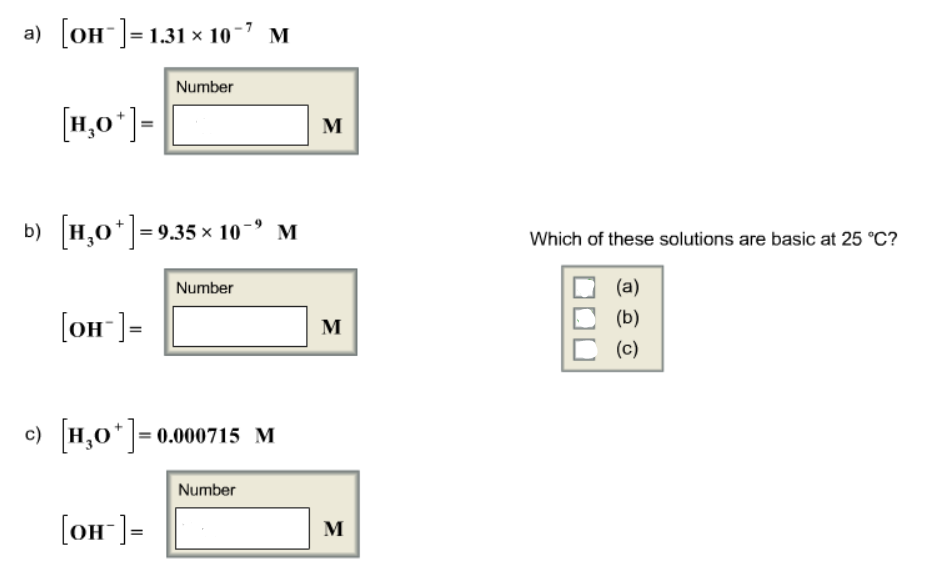# Problem: Calculate either [H3O+] or [OH-] for the solutions below at 25° C

###### FREE Expert Solution

The relationship between [H+]/[H3O+] and [OH-] is connected by the following equation:

$\overline{){{\mathbf{K}}}_{{\mathbf{w}}}{\mathbf{=}}\left[{\mathbf{H}}^{\mathbf{+}}\right]\left[{\mathbf{OH}}^{\mathbf{-}}\right]}$

Kw = autoionization constant of water

Kw = 1.0x10-14 at T = 25°C

a) [OH-] = 1.31 x 10-7 M

[H3O+] = 7.63 x 10-8 M

The [H3O+] of the solution is 7.63 x 10-8 M

80% (388 ratings)###### Problem Details

Calculate either [H3O+] or [OH-] for the solutions below at 25° C